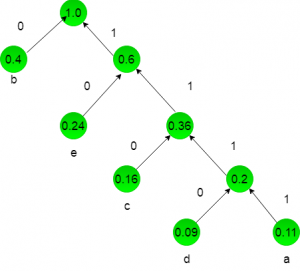# UGC NET COMPUTER SCIENCE SOLVED PAPERS 2017-19 - UGC NET Computer Science November 2017 Paper 3

>>>>>>>>UGC NET Computer Science November 2017 Paper 3

• A

e = i+n• B

e = i+2n• C

e = 2i+n• D

e = 2n+i• A

Ω (n)• B

Ω (n/k)• C

Ω (nlogk )• D

Ω (n/klogn/k)Where b and c are constants. The order of the algorithm corrosponding to above recurrence relation is:

• A

n• B

n2• C

n log n• D

n3• Option : D
• Explanation :
We can use Master theorem to solve this recurrance relation:T(n) = aT(n/2) + Θ(nklogpn)
In given question:
T(n) = 8T(n/2) + Cn
here a = 8 and b = 2 and k = 1.
clearly a > bk
So T(n) = Θ(nlogba )
T(n) = Θ(nlog2 8)
ie T(n) = Θ(n3)
So, option (D) is correct.

• A

5• B

3• C

4• D

2• A

2.40• B

2.16• C

2.26• D

2.15• Option : B
• Explanation :
a = 0.11 b = 0.40 c = 0.16 d = 0.09 e = 0.24 we will draw a huffman tree:now huffman coding for character:
a = 1111, b = 0, c = 110, d = 1111, e = 10
lenghth for each character = no of bits * frequency of occurence:
a = 4 * 0.11 = 0.44
b = 1 * 0.4 = 0.4
c = 3 * 0.16 = 0.48
d = 4 * 0.09 = 0.36
e = 2 * 0.24 = 0.48
Now add these lenght for average length: 0.44 + 0.4 + 0.48 + 0.36 + 0.48 = 2.16